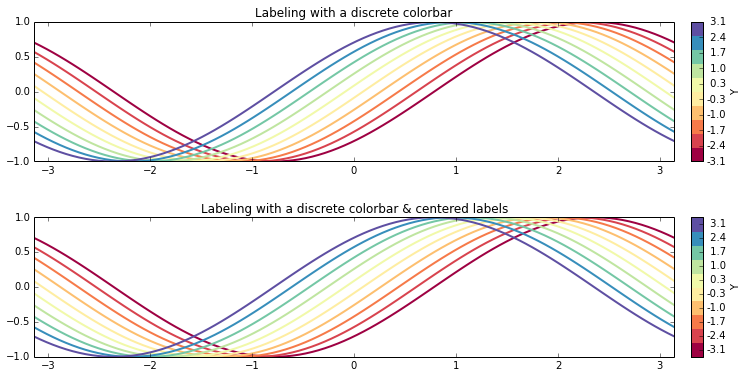The following notebook shows various ways of generating and working with colormaps and functions like pcolormesh() and contourf().

It covers:

• Color and colormap basics
• Generating custom diverging colormaps
• Centering colormaps and displaying the center-valued color
• Differences between pcolormesh() and contourf()
• Example custom colormaps
• Setting out-of-range and bad colors
• Using a colormap to color lines

## Color and colormap basics

### Specifying colors in matplotlib

Most functions that take color arguments (e.g. matplotlib.pyplot.plot) accept the color in a variety of formats. This is done using the method matplotlib.colors.ColorConverter.to_rgba() which converts the color to an RGBA representation, which is a vector of four values from 0-1 specify the Red, Blue, Green, and Alpha channels where 1 is fully-saturated (for RGB) or fully opaque (for Alpha). This RGBA representation is the one used by matplotlib internally.

The various arguments that ColorConverter.to_rgba() can take are:

• a letter from the set ‘rgbcmykw’ (e.g. 'g' for green)
• a hex color string, like ‘#00FFFF’
• an HTML standard name, like ‘aqua’
• a string representation of a float, like ‘0.4’, indicating gray on a 0-1 scale
• an RGB or RGBA vector like [0.5, 0.3, 0.2, 1.0] with each channel scaled in range [0-1]

### Colormaps in matplotlib

The matplotlib.colors.Colormap class is the basis for all colormaps which are just mappings from a scalar value to an RGBA value. This mapping occurs using a couple of different classes: matplotlib.cm.ScalarMappable, matplotlib.colors.Normalize, and matplotlib.colors.Colormap . The primary class is the ScalarMappable. Objects generated by functions like pcolor(), contourf(), scatter(), and imshow() subclass ScalarMappable as a mixin class. Mixin classes provide common functionality, but are not designed to "stand on there own" i.e. they are generally not the primary class of the object. This is what lets the disparate objects like Collection returned by poclor() or scatter() and Image returned by imshow() use the same framework for colormaps.

Each ScalarMappable instance is associated with a Normalize instance and a Colormap instance. The Normalize instance provides the method to scale the data in the ScalarMappable to the range [0,1] while the Colormap maps the [0,1] range to N RGBA colors where N is the size of the colormap. There are two basic types of colormaps included in matplotlib.colors:

• LinearSegmentedColormap: Use a lookup table to interpolate normalized values between given colors.
• ListedColormap: Segmented, discrete colormap using a list of colors.

More information can be found in the matplotlib.colors documentation.

### Default colormaps and names

Matplotlib comes with a large set of default colormaps, illustrated here. Most (all?) of the default colormaps also have a reversed version, given by appending '_r' to its name. These can be retrieved using matplotlib.cm.get_cmap(name) where name is the colormap name. The get_cmap() method recognizes any registered colormaps. To register a colormap, use matplotlib.cm.register_cmap(name, cmap). Note that you do not have to register colormaps to use them, it's just a convenience.

In :
%matplotlib inline
import numpy as np
import matplotlib.pyplot as plt

# Some example default colormaps

# Make up some fake data
x = np.linspace(-np.pi, np.pi, 50)
y = np.linspace(-np.pi, np.pi, 50)
X,Y = np.meshgrid(x,y)
Z = np.sin(X + Y/4)

fig = plt.figure(figsize = (12,2.5))

# Blues
plt.subplot(1,4,1)
plt.pcolormesh(X, Y, Z, cmap=plt.cm.get_cmap('Blues'))
plt.colorbar()
plt.axis([-3, 3, -3, 3])
plt.title('Sequential')

# Red-Blue
plt.subplot(1,4,2)
plt.pcolormesh(X, Y, Z, cmap=plt.cm.get_cmap('RdBu'))
plt.colorbar()
plt.axis([-3, 3, -3, 3])
plt.title('Diverging')

# Red-Blue reversed
plt.subplot(1,4,3)
plt.pcolormesh(X, Y, Z, cmap=plt.cm.get_cmap('RdBu_r'))
plt.colorbar()
plt.axis([-3, 3, -3, 3])
plt.title('Diverging (reversed)')

# Dark2
plt.subplot(1,4,4)
plt.pcolormesh(X, Y, Z, cmap=plt.cm.get_cmap('Dark2'))
plt.colorbar()
plt.axis([-3, 3, -3, 3])
plt.title('Qualatative')

Out:
<matplotlib.text.Text at 0x10986a5d0>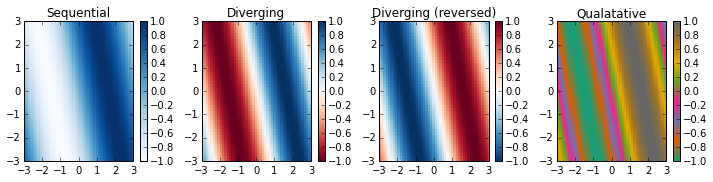## Simple custom colormaps

You can use the LinearSegmentedColormap object to create simple colormaps. While there are a number of ways to generate colormaps with this class, the simplest is to use the method LinearSegmentedColormap.from_list(), passing it a list of colors, a name for the colormap, and the number of levels in the colormap.

In the example below, we use this class within a function that creates a diverging colormap with a low, mid, and high color for a specified number of levels. All colors in between are linearly-interpolated from these values. To see more about this class, check its documentation

In :
def custom_div_cmap(numcolors=11, name='custom_div_cmap',
mincol='blue', midcol='white', maxcol='red'):
""" Create a custom diverging colormap with three colors

Default is blue to white to red with 11 colors.  Colors can be specified
in any way understandable by matplotlib.colors.ColorConverter.to_rgb()
"""

from matplotlib.colors import LinearSegmentedColormap

cmap = LinearSegmentedColormap.from_list(name=name,
colors =[mincol, midcol, maxcol],
N=numcolors)
return cmap

custom_map = custom_div_cmap(11, mincol='g', midcol='0.9' ,maxcol='CornflowerBlue')
plt.pcolormesh(X, Y, Z, cmap=custom_map)
plt.axis([-3, 3, -2, 3])
plt.colorbar()
plt.title('green-gray-blue custom colormap')

Out:
<matplotlib.text.Text at 0x1098e7090>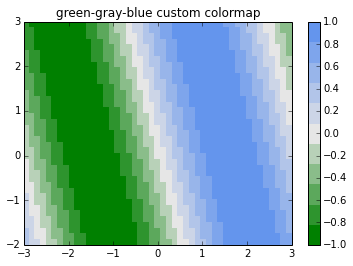## pcolormesh() examples

By default, pcolor() and pcolormesh() use the number of values in the colormap to map the data from the numerical values of the scalar in argument z. To use a different number of colors, change the colormap with the cmap named argument. The number of colors in the colormap will determine the color resolution of the resulting images.

In :
fig = plt.figure(figsize = (12,4))
# Use default bwr colormap
plt.subplot(1,3,1)
plt.pcolormesh(X, Y, Z, cmap=plt.cm.bwr)
plt.colorbar()
plt.axis([-3, 3, -3, 3])
plt.title('Plot demonstrating default \n pcolor interpolation')

#same as above but using a custom colormap with a specified number of colors
#in the colormap
bwr_custom = custom_div_cmap(10)
plt.subplot(1,3,2)
plt.pcolormesh(X, Y, Z, cmap=bwr_custom)
plt.colorbar()
plt.axis([-3, 3, -3, 3])
plt.title('Same as above but using \n a colormap with 10 colors')

#same as above but using a custom colormap with 11 colors to see white
bwr_custom = custom_div_cmap(11)
plt.subplot(1,3,3)
plt.pcolormesh(X, Y, Z, cmap=bwr_custom)
plt.colorbar()
plt.axis([-3, 3, -3, 3])
plt.title('Same, 11 colors \n Notice white in middle')

Out:
<matplotlib.text.Text at 0x109a3ed10>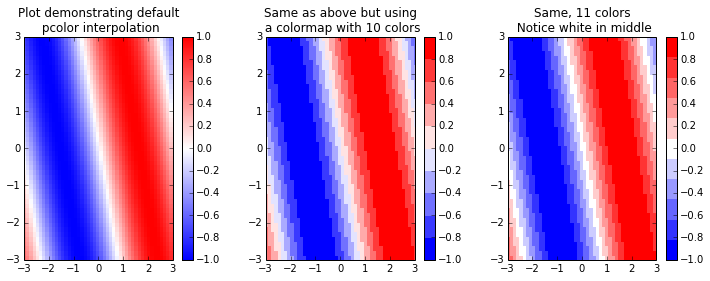## Setting color limits, levels, and coloring around zero

With diverging colormaps, you're often trying to show values that positively or negatively diverge from a central tendency or zero value, and, therefore it is important to make sure that the desired zero-color (usually white, light gray, or yellowish) is displayed correctly. First, and most importantly, is to set the colorlimits, variously called vmin/vmax or clim. There are a few ways to do so:

• Set the vmin and vmax arguments in the call to pcolor(), pcolormesh(), contourf(), or other plotting function.
• You can also set the clim argument (like below) in the call to the plotting method.
• Use plt.clim(vmin, vmax) or plt.clim([vmin,vmax]) to set the limits of the current image.
• Use ScalarMappable.set_clim(). This uses the object's method to set the limits. For example:
  pc_obj = pcolormesh(z)
pc_obj.set_clim(-0.5, 0.5)


All of these methods set the upper and lower limits of the matplotlib.colors.Normalize instance that is used to normalize the ScalarMappable values to the range 0-1. Essentially, these are the upper and lower limits of the colormap. By setting these values such that the are equivalent around zero, your colormap will be centered where you intend it. In general, for a linear mapping, the middle color will be located at a value of (vmax-vmin)/2, i.e. the midpoint or average of vmin and vmax.

In :
# Uncentered colormap
fig, ax = plt.subplots(1,2, figsize=(12,4))
cm = plt.cm.bwr

pc0 = ax.pcolormesh(X, Y, Z, cmap=cm, vmin=-1, vmax=1.5)
plt.colorbar(pc0,ax=ax)
ax.axis([-3, 3, -3, 3])
ax.set_title('Un-centered colormap')

# Centered colormap
pc1 = ax.pcolormesh(X, Y, Z, cmap=cm, vmin=-1.5, vmax=1.5)
plt.colorbar(pc1, ax=ax)
ax.axis([-3, 3, -3, 3])
ax.set_title('Centered colormap')

Out:
<matplotlib.text.Text at 0x103fc3590>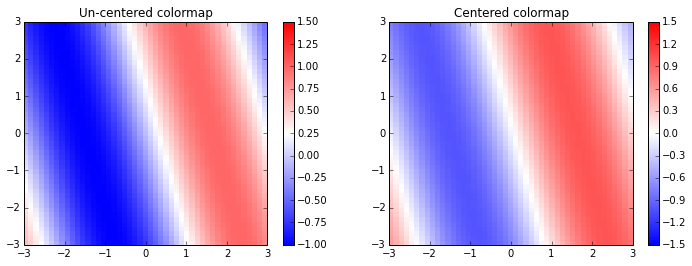### Difference between contourf and pcolormesh

One thing to be aware of when using this limits, however, is how contourf() and pcolormesh() differ using clim or vmin/vmax. With pcolormesh(), the colormap limits will always be set based on the clim values. With contourf(), if clim or vmin/vmax values are given without contour levels, the levels will be automatically chosen, but the color limits will be correct. For example, if your data goes outside the clim range, the default colorbar will too, but all the levels outside the color limits will still be drawn - they will just be the same color.

On the other hand, if both clim or vmin/vmax and the contour levels are given, only the contour levels are obeyed and the clim values are ignored. See examples below, but some testing still needed to confirm this status...

In :
# Show variations in contourf, levels, and limits.  Also so alternate clim-setting methods
fig, ax = plt.subplots(1,4, figsize=(15,4))
cm = plt.cm.bwr

# Limits only, inside data range
pc0 = ax.contourf(X, Y, Z, cmap=cm)
pc0.set_clim(-0.5,0.5)
plt.colorbar(pc0,ax=ax)
ax.axis([-3, 3, -3, 3])
ax.set_title(u'Limits only: ±0.5')

# Limits only, outside data range
pc1 = ax.contourf(X, Y, Z, cmap=cm, vmin=-1.5, vmax=1.5)
plt.colorbar(pc1,ax=ax)
ax.axis([-3, 3, -3, 3])
ax.set_title(u'Limits only: ±1.5')

# Limits and levels
clvls = np.linspace(-1,1,10)
pc2 = ax.contourf(X, Y, Z, clvls, cmap=cm, clim=[-2, 2])
plt.colorbar(pc2, ax=ax)
ax.axis([-3, 3, -3, 3])
ax.set_title(u'Limits (±2) and levels')

# Levels only
pc3 = ax.contourf(X, Y, Z, clvls, cmap=cm)
plt.colorbar(pc3, ax=ax)
ax.axis([-3, 3, -3, 3])
ax.set_title('Levels only')

Out:
<matplotlib.text.Text at 0x109c67190>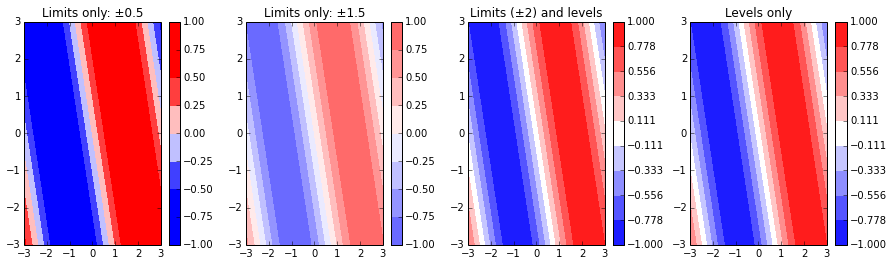### Displaying the midpoint color

Whether the midpoint color is actually displayed depends on the number of colors in the colormap and/or the plotting function used. In general, an even number of colors splits the zero value (it becomes an edge) while and odd number will display the mid-range color. Because contourf() plots level end-points, you need one extra level or color than when using pcolormesh().

The rules break down as follows:

• Display mid-color:

• Odd colormap size: pcolor()
• Even number of levels: contourf()
• Mid-color as 'edge':

• Even colormap size: pcolor()
• Odd number of levels: contourf()
In :
# Show variations in centering-on-white
fig, ax = plt.subplots(2,2, figsize=(10,6))
fig.suptitle('Differences in pcolor, contourf, and white in center')

# Pcolor odd
a = ax[0,0]
pc = a.pcolormesh(X, Y, Z, cmap=custom_div_cmap(11), clim=(-1,1))
plt.colorbar(pc,ax=a)
a.axis([-3, 3, -3, 3])
a.set_title(u'Pcolor, odd colormap')

# Pcolor even
a = ax[0,1]
pc = a.pcolormesh(X, Y, Z, cmap=custom_div_cmap(10), clim=(-1,1))
plt.colorbar(pc,ax=a)
a.axis([-3, 3, -3, 3])
a.set_title(u'Pcolor, even colormap')

# Contourf odd levels
clvls = np.linspace(-1,1,11)
a = ax[1,0]
pc = a.contourf(X, Y, Z, clvls, cmap=custom_div_cmap(11))
plt.colorbar(pc,ax=a)
a.axis([-3, 3, -3, 3])
a.set_title(u'contourf, odd levels')

# Contourf odd levels
# Note: same colormap is used as before
clvls = np.linspace(-1,1,10)
a = ax[1,1]
pc = a.contourf(X, Y, Z, clvls, cmap=custom_div_cmap(11))
plt.colorbar(pc,ax=a)
a.axis([-3, 3, -3, 3])
a.set_title(u'contourf, even levels')

Out:
<matplotlib.text.Text at 0x104449610>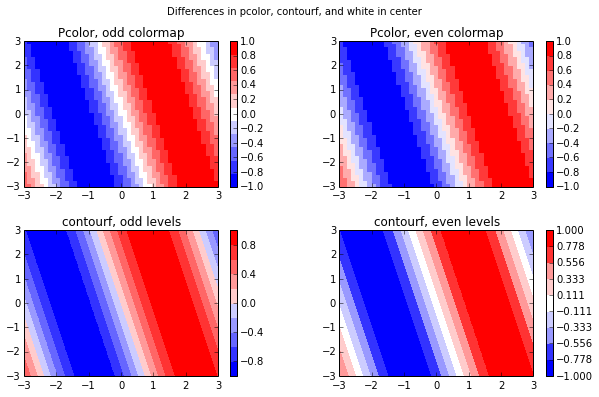### Over-laying a zero-line

Alternatively, you can just plot a contour-level at 0 on top of your main figure by using the V argument of contour().

In :
# Show variations with contour lines overlaid
fig, ax = plt.subplots(2,2, figsize=(10,6))
fig.suptitle('Using overlaid contour lines')

# Pcolor odd
a = ax[0,0]
pc = a.pcolormesh(X, Y, Z, cmap=custom_div_cmap(11), clim=(-1,1))
a.contour(X,Y,Z,,colors='0.4', linewidths=1)
plt.colorbar(pc,ax=a)
a.axis([-3, 3, -3, 3])
a.set_title(u'Pcolor, odd colormap')

# Pcolor even
a = ax[0,1]
pc = a.pcolormesh(X, Y, Z, cmap=custom_div_cmap(10), clim=(-1,1))
a.contour(X,Y,Z,,colors='0.4', linewidths=1)
plt.colorbar(pc,ax=a)
a.axis([-3, 3, -3, 3])
a.set_title(u'Pcolor, even colormap')

# Contourf odd levels
clvls = np.linspace(-1,1,11)
a = ax[1,0]
pc = a.contourf(X, Y, Z, clvls, cmap=custom_div_cmap(11))
a.contour(X,Y,Z,,colors='0.4', linewidths=1)
plt.colorbar(pc,ax=a)
a.axis([-3, 3, -3, 3])
a.set_title(u'contourf, odd levels')

# Contourf odd levels
# Note: same colormap is used as before
clvls = np.linspace(-1,1,10)
a = ax[1,1]
pc = a.contourf(X, Y, Z, clvls, cmap=custom_div_cmap(11))
a.contour(X,Y,Z,,colors='0.4', linewidths=1)
plt.colorbar(pc,ax=a)
a.axis([-3, 3, -3, 3])
a.set_title(u'contourf, even levels')

Out:
<matplotlib.text.Text at 0x1046a94d0>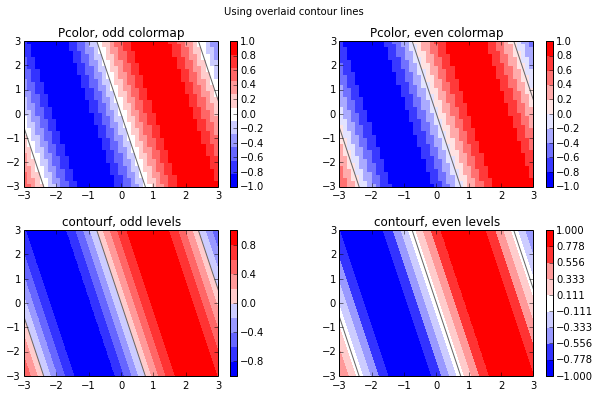### Big and small colormaps with contourf

Also note that even if you're using contourf() with an even number of levels, your colormap still needs to resolve the central color for you to see it. This is true even though not all levels of the colormap are displayed on the colorbar (all contourf() levels are displayed though). This means you either need:

• Odd-length colormap: The middle color is explicitly resolved.
• Large, even or odd colormap: As the colormap size gets larger, the resolution around the central point gets greater. Even if the map is even and doesn't explicitly resolve the central color, you'll get pretty close, although it probably wont' be perfect.

What won't work is a small, even colormap. There's so few colors that the central, neutral color will likely not be well-resolved.

In :
# Resolving white
fig, ax = plt.subplots(1,3, figsize=(14,4))

# Contourf even, few colors w/ white
clvls = np.linspace(-1,1,10)
a = ax
pc = a.contourf(X, Y, Z, clvls, cmap=custom_div_cmap(11))
plt.colorbar(pc,ax=a)
a.axis([-3, 3, -3, 3])
a.set_title(u'even levels / small, odd colormap')

# Contourf even, few colors
clvls = np.linspace(-1,1,10)
a = ax
pc = a.contourf(X, Y, Z, clvls, cmap=custom_div_cmap(10))
plt.colorbar(pc,ax=a)
a.axis([-3, 3, -3, 3])
a.set_title(u'even levels / small, even colormap')

# Contourf even, many colors
# Note: same colormap is used as before
clvls = np.linspace(-1,1,10)
a = ax
pc = a.contourf(X, Y, Z, clvls, cmap=custom_div_cmap(100))
plt.colorbar(pc,ax=a)
a.axis([-3, 3, -3, 3])
a.set_title(u'even levels / large, even colormap')

Out:
<matplotlib.text.Text at 0x1048ec410>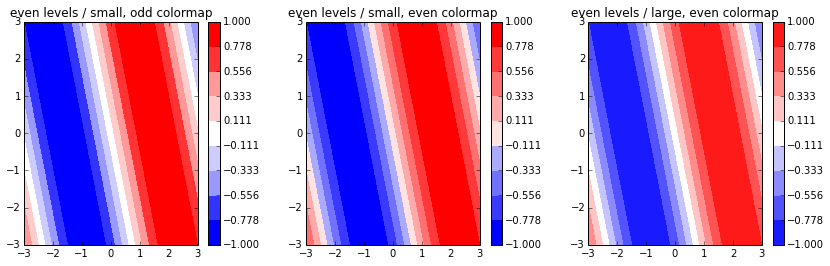## Custom colormap examples from ColorBrewer

We now use our custom_div_cmap() function to show some examples colormaps. These examples were dervied from Colorbrewer which has some great examples of various colormaps.

In :
# Define a function to do each plot.  Note the use of the **div_cmap_args to
# pass extra arguments to the custom_div_cmap function.

def cmap_example_plot(x, y, z, title='blue-white-red', **div_cmap_args):
plt.pcolormesh(x, y, z, cmap=custom_div_cmap(**div_cmap_args))
plt.colorbar()
plt.axis([-3, 3, -2, 3])
plt.title(title)

# Create a figure object, title it, and do the plots.
fig = plt.figure(figsize = (9,6))
fig.suptitle('Example colormaps')

plt.subplot(2,2,1)
cmap_example_plot(X, Y, Z, title='green-white-purple',
numcolors=21, mincol='#1b7837', maxcol='#762a83')

plt.subplot(2,2,2)
cmap_example_plot(X, Y, Z, title= 'teal-white-brown',
numcolors=21, mincol='#01665e', maxcol='#8c510a')

plt.subplot(2,2,3)
cmap_example_plot(X, Y, Z, title='grey-white-red',
numcolors=21, mincol='#4d4d4d', maxcol='#b2182b')

plt.subplot(2,2,4)
cmap_example_plot(X, Y, Z, title='green-yellow-red/orange',
numcolors=21, mincol='#1a9641', maxcol='#d73027', midcol='#ffffbf')

plt.show()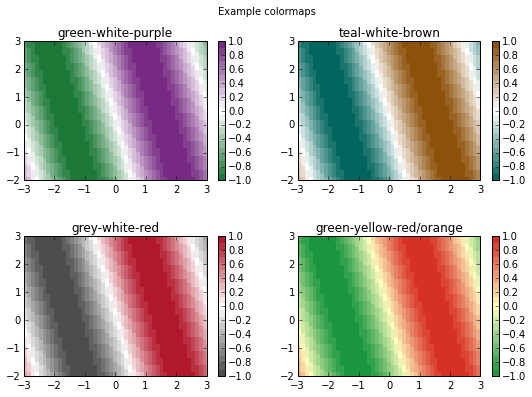## Setting upper, lower, and bad values

Each colormap instance also has three special values that can be used to display data outside of the normalized [0,1] range or for bad (masked) data. These can be set by either manipulating the colormap object or using extend argument with contourf() or plt.colorbar(). The extend argument is nice because it automatically uses the given colormap for the out-of-range colors whereas you have explicitly set these colors with the colormap methods, however using the colormap methods is the only option when plotting with pcolormesh().

• Colormap functions:
• Colormap.set_under(color) Set low out-of-range color.
• Colormap.set_over(color) Set high out-of-range color.
• Colormap.set_bad(color) Set masked-data color.
• contourf() and pyplot.colorbar() extend argument:
• contourf(..., extend='neither') Do not extend contour levels (default).
• contourf(..., extend='min') Extend low out-of-range.
• contourf(..., extend='max') Extend high out-of-range.
• contourf(..., extend='both') Extend low and high out-of-range

Note that for the out-of-range values to display on the colorbar, you have to use the extend keyword argument, e.g.: pyplot.colorbar(extend='both')

Also note that contouring excludes masked regions entirely and therefore colormap.set_bad() has no effect.

In :
# Pcolor with upper/lower/bad values

# Use the green-yellow-red/orange from above
cmap = custom_div_cmap(numcolors=51, mincol='#1a9641', maxcol='#d73027', midcol='#ffffbf')

# Set some garish out-of-range colors and gray for bad
cmap.set_under('DeepPink')
cmap.set_over('LawnGreen')

# Copy Z and add some bad data points around +/- 0.5
bad = np.logical_and(np.abs(Z) > 0.4, np.abs(Z) < 0.6)

# Setup the fig and do the pcolor plot
fig, ax = plt.subplots(1,2, figsize=(12,4))
pc0 = ax.pcolormesh(X, Y, Z2, cmap=cmap, vmin=-0.9, vmax=0.9)
plt.colorbar(pc0,ax=ax, extend='both')
ax.axis([-3, 3, -3, 3])

# Do the contourf version using teal-white-brown from above
cmap2 = custom_div_cmap(numcolors=51, mincol='#01665e', maxcol='#8c510a')

# Just set bad and let contourf do the rest.  Note it doesn't do anything.

pc1 = ax.contourf(X, Y, Z2, 20, cmap=cmap2, vmin=-0.9, vmax=0.9, extend='both')
plt.colorbar(pc1, ax=ax)
ax.axis([-3, 3, -3, 3])

Out:
<matplotlib.text.Text at 0x10c340690>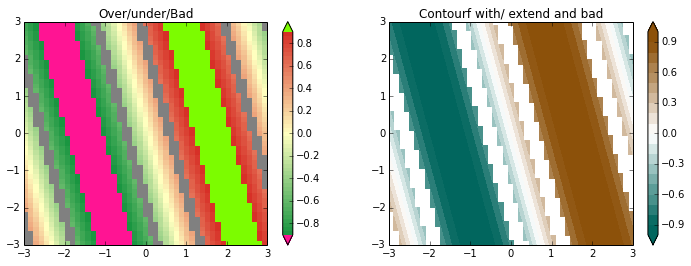# Generating line colors with a colormap

Generating lines with a colormap can be done by calling the colormap as a method: colormap(x). x is an numpy array of either float or int. For floats, x should be in the range [0,1] whereas for integers x should be in [0, colormap.N) and x indexes directly into the colormap. This method returns a 4-tuple of RGBA values if x is scalar, otherwise it returns an array of shape x.shape + (4,).

To label these plots, you can either use the label argument and a legend, or generate a colorbar. Colorbar generation is a bit more difficult, however, as you have to create a ScalarMappable object to use the pyplot.colorbar() method. If you use a large enough colormap with enough steps, the colorbar will appear continuous.

A more pythonic way of generating this type of plot might be to use a matplotlib.collections.LineCollection object which includes a ScalarMappable. I found this just as cumbersome as the method below. Perhaps someone could write a function, say plot_colormap_lines() that correctly creates the LineCollection object and returns it such that this type of plot is just as easy as plt.plot()...

In :
# Use the spectral colormap for examples
cmap = plt.cm.Spectral

# Generate some fake data
N = 100
nlines = 10
x = np.linspace(-np.pi, np.pi, N)
y = np.linspace(-np.pi, np.pi, nlines)

# Use np.newaxis to create [N,1] and [1,Nlines] x and y arrays
# Then broadcasting to generate Z with shape [N,Nlines]
z = np.sin(x[:,np.newaxis] + y[np.newaxis,:]/4)

# Use 0-1 values to generate the colors with the linspace method
line_colors = cmap(np.linspace(0,1,nlines))

# We have to generate our own axis to put the colorbar in
# otherwise it "steals" space from the current axis.  Please
# let me know if anyone has found another way around this,
# because the custom axes generation is the only way I've
# figured out.
from matplotlib.gridspec import GridSpec
fig = plt.figure(figsize = (12,6))
nrows = 2
gs = GridSpec(nrows,2,width_ratios=[50,1])
ax = [plt.subplot(gs[i,0]) for i in range(nrows)]
cbax1 = plt.subplot(gs[1,1])

# First, plot lines w/ legend
a = ax
a.set_title('Labeling with a legend')

for i in range(nlines):
a.plot(x, z[:,i], c=line_colors[i],lw=3,label='{:4.1f}'.format(y[i]))
leg = a.legend(loc='center left', bbox_to_anchor=(1, 0.5), ncol=2)
leg.set_title('Y')

# Next, plot with colorbar
a = ax
a.set_title('Labeling with a "continuous" colorbar')

for i in range(nlines):
a.plot(x, z[:,i], c=line_colors[i],lw=3,label='{:3.1f}'.format(y[i]))

# Generate fake ScalarMappable for colorbar
sm = plt.cm.ScalarMappable(cmap=cmap, norm=plt.Normalize(vmin=y,vmax=y[-1]))
sm.set_array([])  # You have to set a dummy-array for this to work...
cbar = plt.colorbar(sm, cax=cbax1)
cbar.set_label('Y')
cbar.set_ticks(y)
cbar.set_ticklabels(['{:4.1f}'.format(yi) for yi in y]) # Make 'em nicer-looking

# Moves colorbar closer to main axis by adjusting width-spacing between subplot axes.

# Set axis limits
for a in ax:
a.set_xlim(-np.pi, np.pi)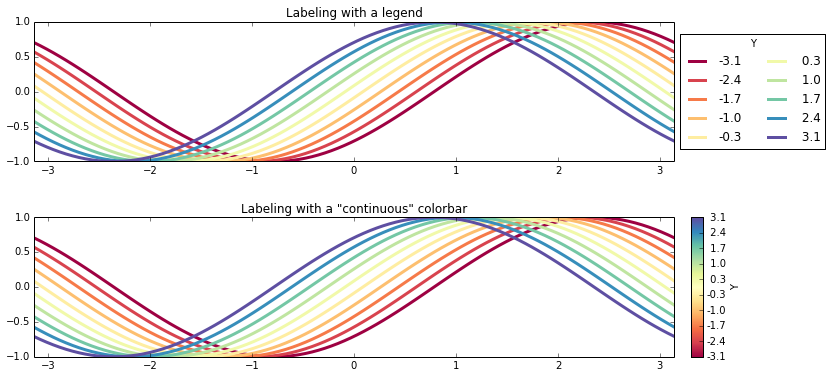Because you're only drawing a few lines, you may wish to use a discrete colormap, with only as many divisions as lines. If you use a smaller colormap, say with only the same number of elements as lines you are plotting, the labeling of the colormap will be off a bit. While the colors and labels are correct, because the endpoints define the colormap and colorbar boundaries, the position of the ticks for each color will vary i.e. the ticks will not be centered in the color.

If you look at the top panel in the example below, you'll see that the lower-most tick is at the bottom of the color patch and as you move up the colorbar, the ticks move from the lower end of the patch to the upper end of the patch. At the upper-most patch, the tick is at the top edge of the box. This is because the colorbar function doesn't know how to extend the patches at the top and bottom edges, and thus uses those as the boundaries.

To center the labels, you can provide a boundaries argument to the colorbar() function. You can generate this by using numpy.linspace(vmin-dy/2, vmax+dy/2, Nlines+1) where dy is the spacing between the values being plotted.

In :
fig = plt.figure(figsize = (12,6))
nrows = 2
gs = GridSpec(nrows,2,width_ratios=[50,1])
ax = [plt.subplot(gs[i,0]) for i in range(nrows)]
cbax = [plt.subplot(gs[i,1]) for i in range(nrows)]

# We'll use the same fake ScalarMappable and colormap for each example
from matplotlib.colors import  ListedColormap
cmap2 = ListedColormap(line_colors)
sm = plt.cm.ScalarMappable(cmap=cmap2,
norm=plt.Normalize(vmin=y,vmax=y[-1]))
sm.set_array([])

# Discrete colorbar with default spacing
a = ax
a.set_title('Labeling with a discrete colorbar')

for i in range(nlines):
a.plot(x, z[:,i], c=line_colors[i],lw=2,label='{:4.1}'.format(y[i]))

cbar = plt.colorbar(sm, cax=cbax)
cbar.set_label('Y')
cbar.set_ticks(y)
cbar.set_ticklabels(['{:4.1f}'.format(yi) for yi in y]) # Make 'em nicer-looking

# Discrete colorbar with centered ticks
a = ax
a.set_title('Labeling with a discrete colorbar & centered labels')

for i in range(nlines):
a.plot(x, z[:,i], c=line_colors[i],lw=2,label='{:4.1}'.format(y[i]))

# Generate custom bounds so that ticks are centered
dy = y-y
ybounds = np.linspace(y-dy/2., y[-1]+dy/2., nlines+1)
cbar = plt.colorbar(sm, cax=cbax, boundaries=ybounds)
cbar.set_label('Y')
cbar.set_ticks(y)
cbar.set_ticklabels(['{:4.1f}'.format(yi) for yi in y]) # Make 'em nicer-looking

# Set axis limits
for a in ax:
a.set_xlim(-np.pi, np.pi)

# Moves colorbar closer to main axis by adjusting width-spacing between subplot axes.